Question

# How to isolate velocity [ v ] in the following equation: K ( e2 / r2...

How to isolate velocity [ v ] in the following equation:

K ( e2 / r2 ) = M ( v2 / r )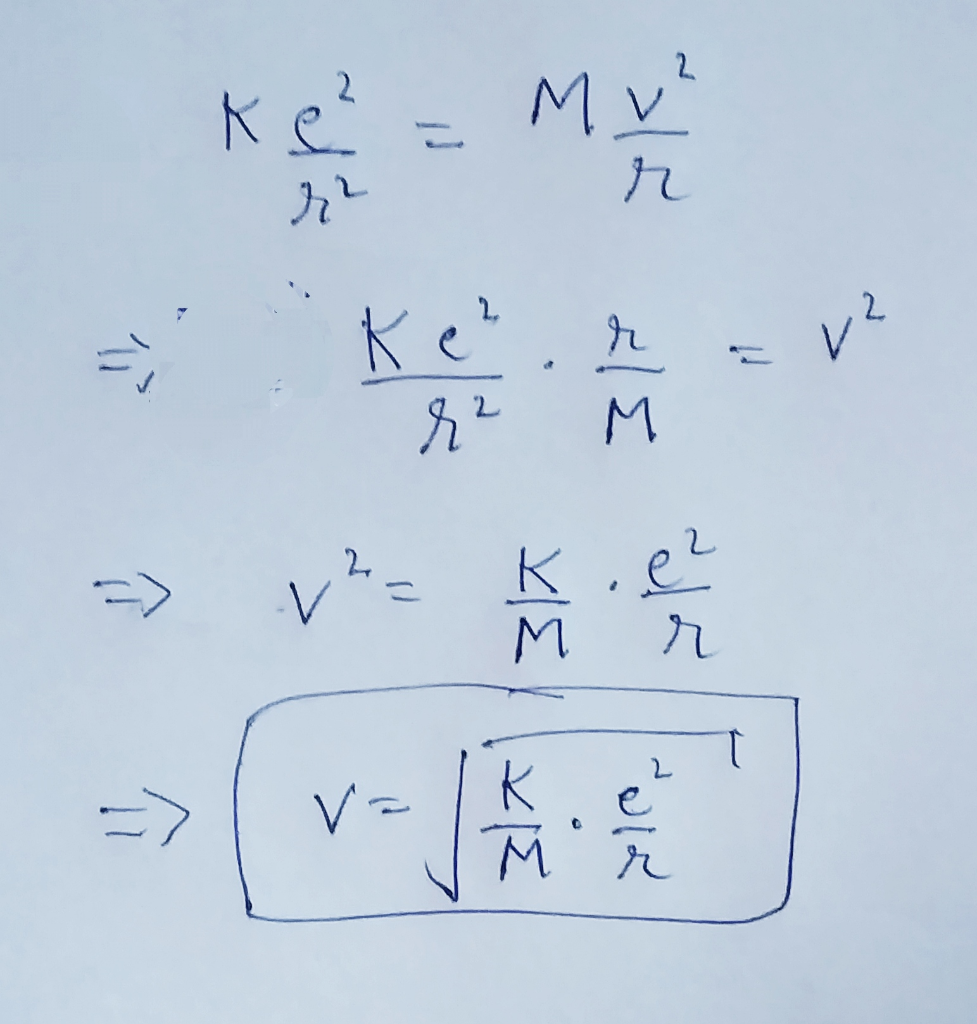#### Earn Coins

Coins can be redeemed for fabulous gifts.

Similar Homework Help Questions
• ### For the above circuit, E1 = 3.57 V, E2 = 5.79 V. r1 = 0.591 Ω, r2 = 0.781 Ω, R1 = 41.3 Ω, R2 = 65...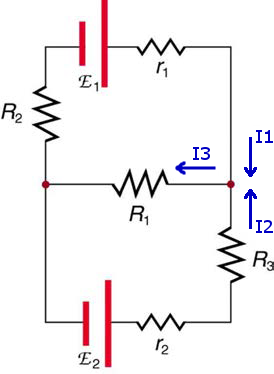For the above circuit, E1 = 3.57 V, E2 = 5.79 V. r1 = 0.591 Ω, r2 = 0.781 Ω, R1 = 41.3 Ω, R2 = 65.1 Ω, R3 = 73.3 Ω. a)Which equation would describe Kirchhoff's Junction Law at the right junction? A I1 - I2 = I3 B  I1 + I2 = I3 C I1 + I3 = I2 D I1 = I2 + I3 b)Which equation would describe Kirchhoff's Loop Law for the lower half-loop? A E2...

• ### 3.) A mechanism has the following velocity equation. where r3 and θ4 are constants and θ,...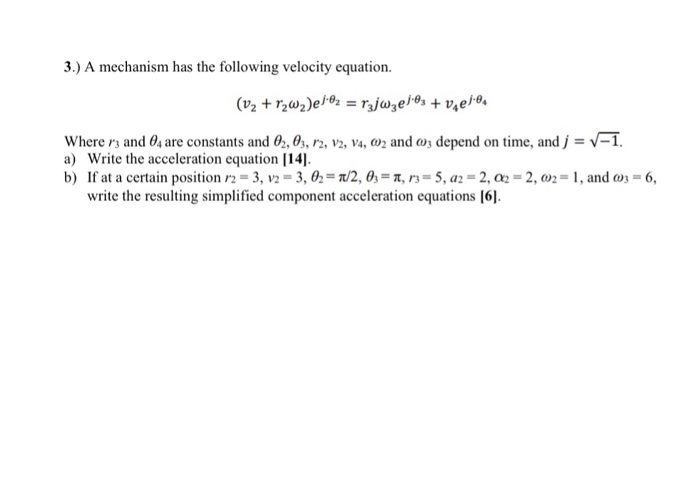3.) A mechanism has the following velocity equation. where r3 and θ4 are constants and θ, θ, r2, V2, V4, (02 and depend on time, and j-V-1. a) Write the acceleration equation 14]. b) If at a certain position r2-3, V2 = 3.A= π/2, 0.-a, n-5, a2-2, o2-2, an= 1, and ω3-6, write the resulting silieceleationeuaions l6l

• ### For the following multistage amplifier, find V2 if Vo=40 V, V1=0.2 V, R1=12.0 K2, R2=9.0 KQ,...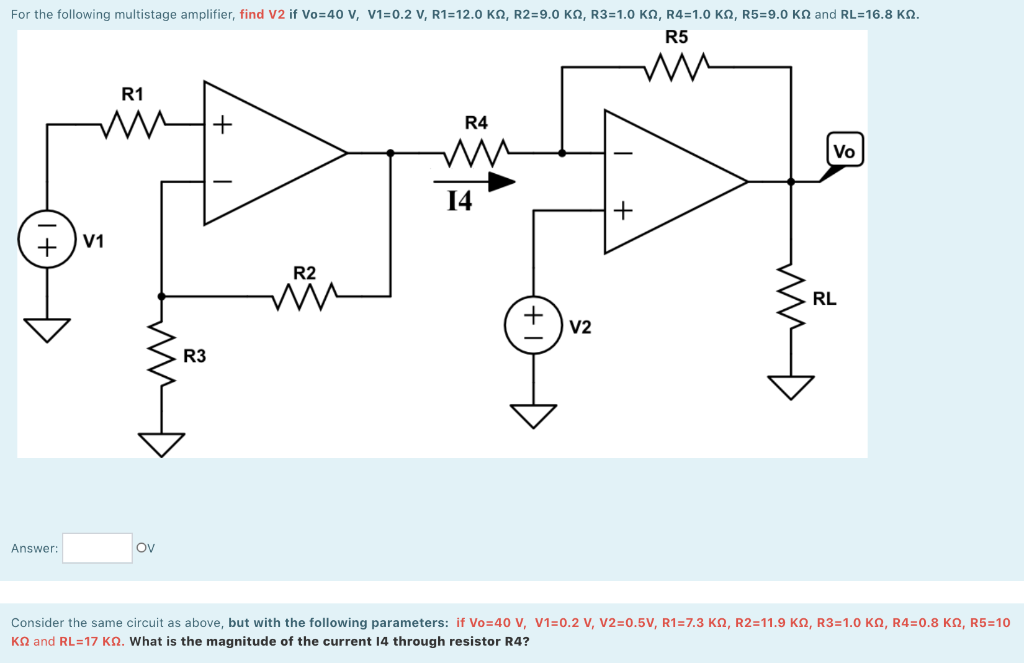For the following multistage amplifier, find V2 if Vo=40 V, V1=0.2 V, R1=12.0 K2, R2=9.0 KQ, R3=1.0 K, R4=1.0 K2, R5=9.0 K and RL=16.8 K. R5 R1 + R4 Vo 14 V1 R2 RL + V2 no R3 Answer: OV Consider the same circuit as above, but with the following parameters: if Vo=40 V, V1=0.2 V, V2=0.5V, R1=7.3 K2, R2=11.9 K2, R3=1.0 K, R4=0.8 K2, R5=10 K2 and RL=17 K2. What is the magnitude of the current 14 through resistor...

• ### The emfs in the figure below are &1 = 5.00 V and E2 = 18.0 V....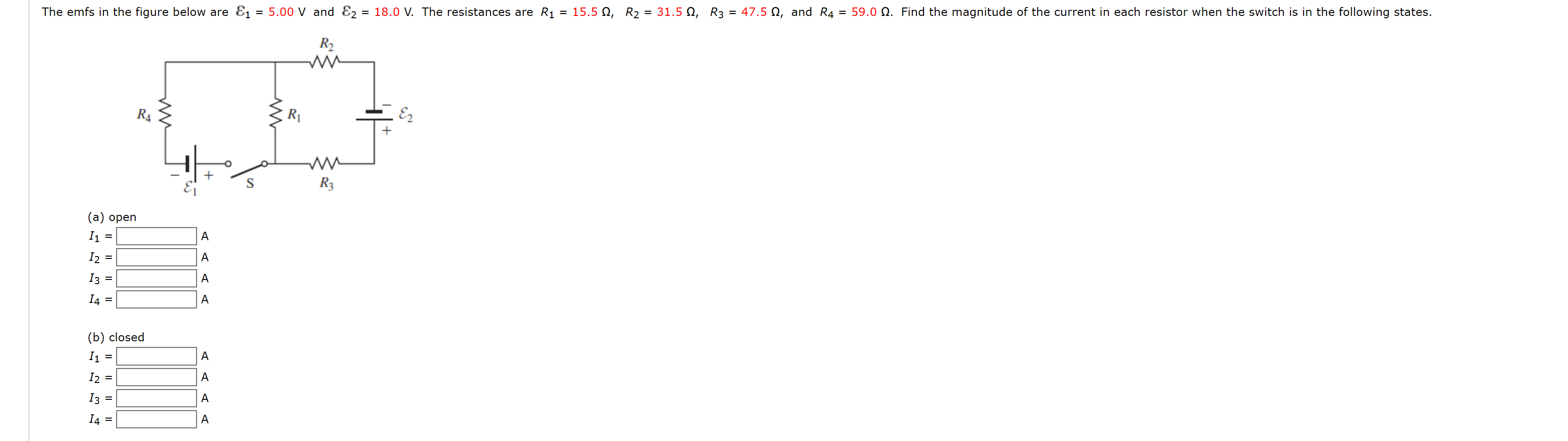The emfs in the figure below are &1 = 5.00 V and E2 = 18.0 V. The resistances are Ri = 15.5 12, R2 = 31.5 12, R3 = 47.5 12, and R4 = 59.0 12. Find the magnitude of the current in each resistor when the switch is in the following states. R2 R4 R E2 R3 A (a) open 11 = 12 = 13 14 = A A A A (b) closed 11 12 13 14 = =...

• ### Dimensional analysis: show that each of following equations is dimensionally correct or incorrect x=distance, v=velocity, v.=velocity,...

Dimensional analysis: show that each of following equations is dimensionally correct or incorrect x=distance, v=velocity, v.=velocity, a=acceleration, t=time 1. x=vt2-at 2. v2=2ax-v2 3. x=v2/4a 4. V=1/3pieR3h where V=volume of a conical shape, pie=con tan t, R=radius, and h=height

• ### Please solve the following problem. 6-33 In Fig. 6-43, solve for , R R2, V2, V,...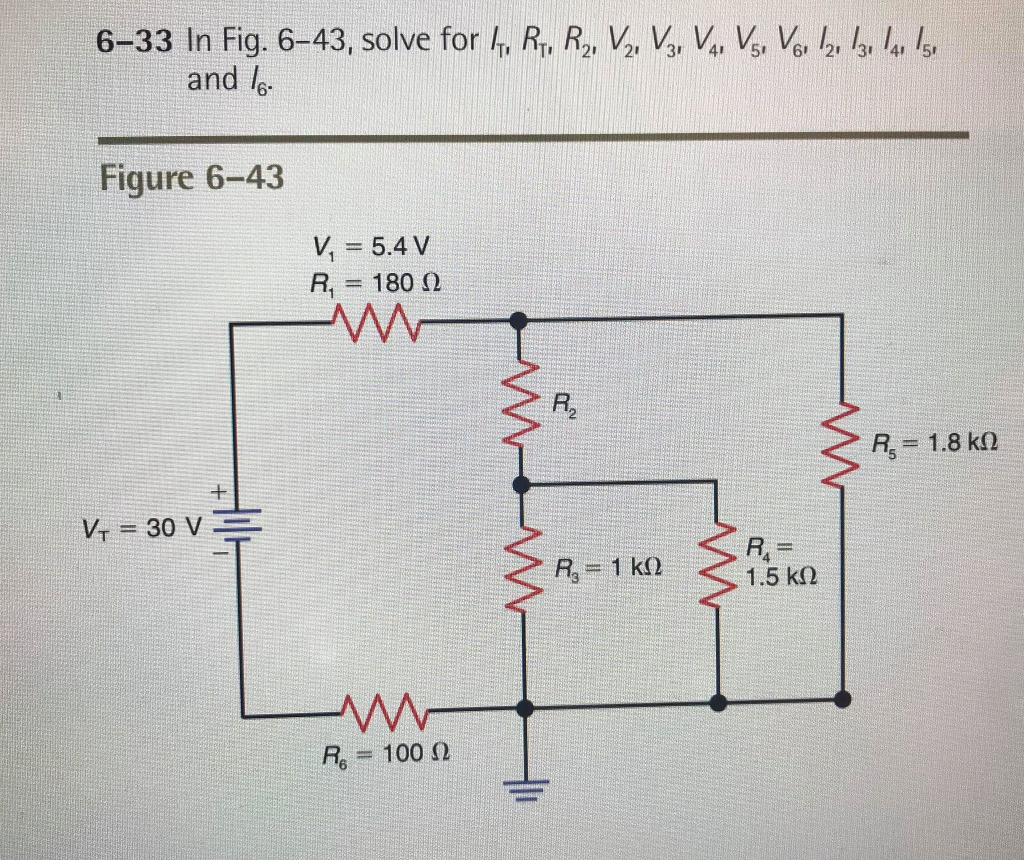Please solve the following problem. 6-33 In Fig. 6-43, solve for , R R2, V2, V, V, , Vo, 2 /3 l 5 and l Figure 6-43 V,=5.4 V R1 = 180 Ω P/2 K. = 30 V 4 1.5 kΩ Re-100 Ω

• ### The emfs in the figure below are &1 = 7.00 V and E2 = 18.0 V....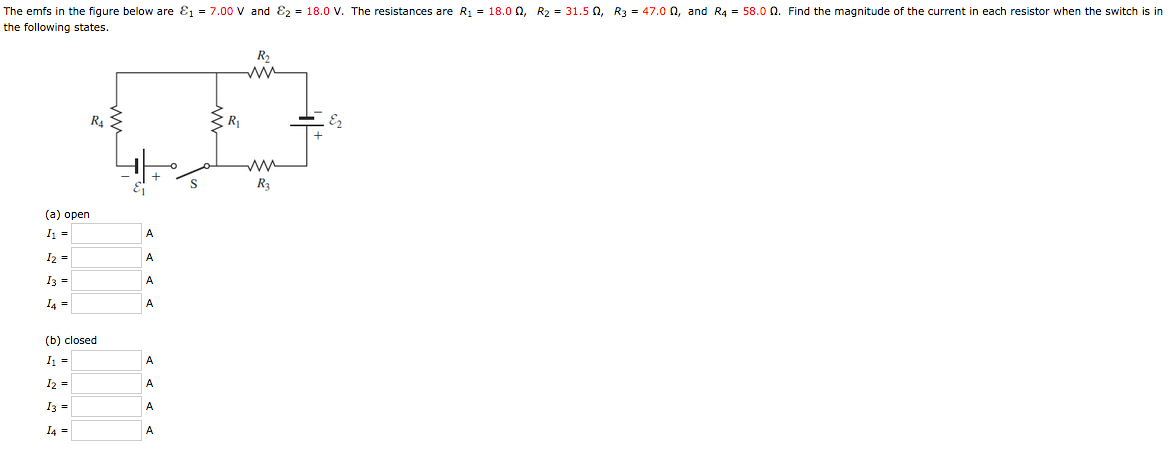The emfs in the figure below are &1 = 7.00 V and E2 = 18.0 V. The resistances are R1 = 18.0 , R2 = 31.5 , R3 = 47.00, and R4 = 58.0 N. Find the magnitude of the current in each resistor when the switch is in the following states. w R\$ 3 R w R3 (a) open 11 = A A 12 = 13 = A A (b) closed 11 А A 12 = 13 = 14...

• ### 3) The velocity v(t) of a skydiver falling to the ground is governed by the equation...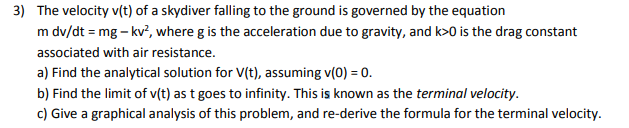3) The velocity v(t) of a skydiver falling to the ground is governed by the equation m dv/dt mg-kv, where g is the acceleration due to gravity, and k>0 is the drag constant associated with air resistance a) Find the analytical solution for V(t), assuming v(0) 0 b) Find the limit of v(t) as t goes to infinity. This is known as the terminal velocity. c) Give a graphical analysis of this problem, and re-derive the formula for the terminal...

• ### Is = 2 mA , V , = 6 V , Ri = 1.5 k 2...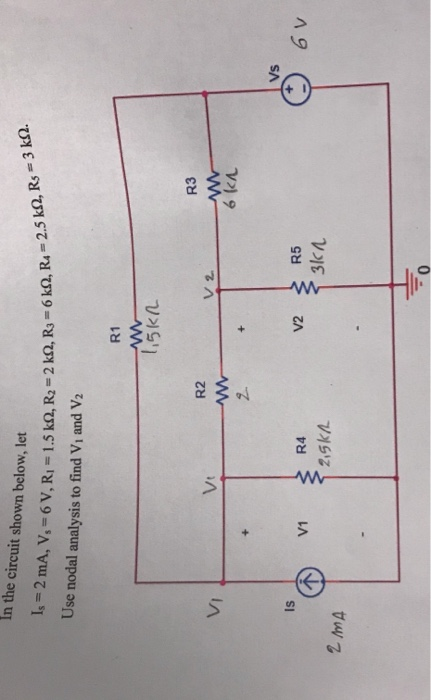Is = 2 mA , V , = 6 V , Ri = 1.5 k 2 , R2 = 2 k2 , R3 = 6k02 , R4 = 2.5 k - 2 , Rs = 3 k12 . In the circuit shown below, let Use nodal analysis to find V and V2 R1 1,5kn VI V va R2 w 2 R3 w 6kA + Vs Is V1 R4 V2 R5 WW 6v 2,5kn 3lk / 2 MA

• ### The emfs in the figure below are &z = 5.00 V and E2 = 21.0 V....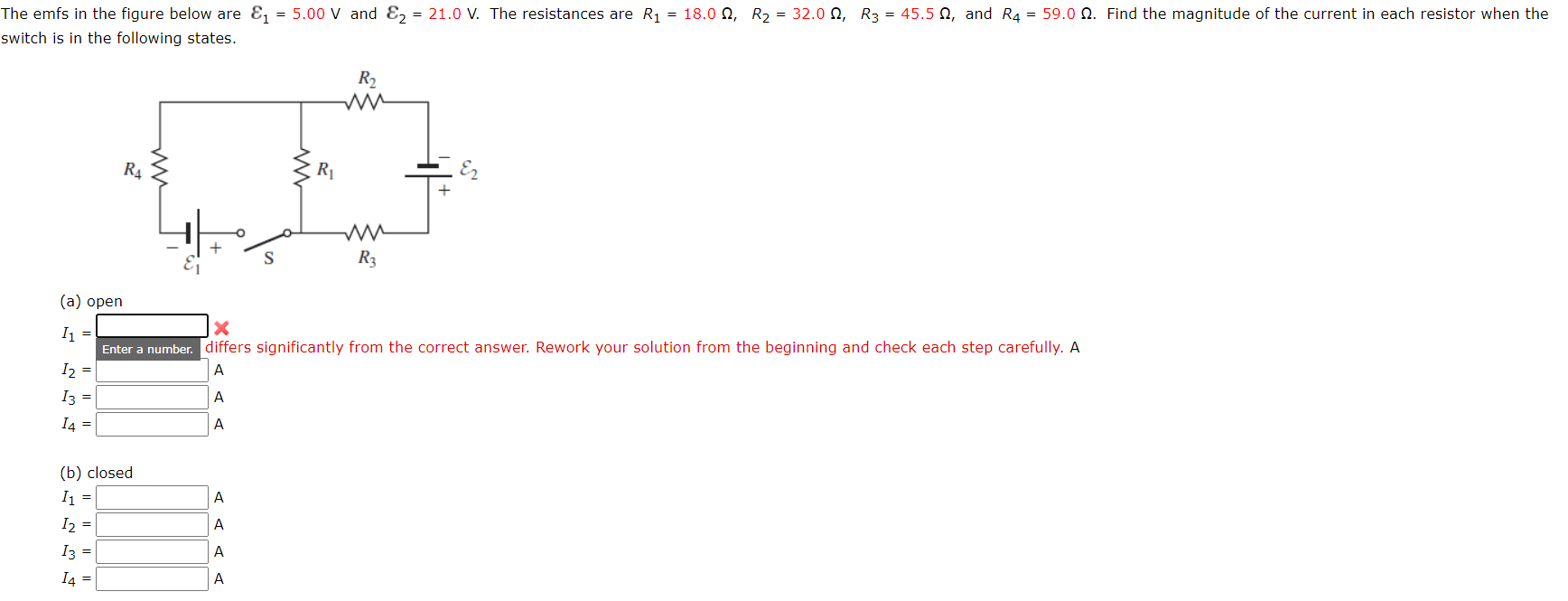The emfs in the figure below are &z = 5.00 V and E2 = 21.0 V. The resistances are R1 = 18.0 , R2 = 32.00, R3 = 45.5 2, and R4 = 59.0 9. Find the magnitude of the current in each resistor when the switch is in the following states. R2 R4 R S R3 (a) open 11 = X Enter a number. differs significantly from the correct answer. Rework your solution from the beginning and check each...